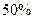Chapter 28, Problem 41P### Principles of Economics 2e

2nd Edition
Steven A. Greenlaw; David Shapiro
ISBN: 9781947172364

#### Solutions

Chapter
Section### Principles of Economics 2e

2nd Edition
Steven A. Greenlaw; David Shapiro
ISBN: 9781947172364
Textbook Problem

# Suppose now that economists expect the velocity of money to increase byas a result of the monetary stimulus. What will be the total Increase in nominal GDP?

To determine

The change in nominal GDP, given change in money supply and the increase in the velocity of money by 50%.

Explanation

The velocity of money is pace of circulation of money in the economy. The formula for calculating velocity of money is given below.

velocityofmoney=Nominal GDPMoneySupplyv=YMS

Given Information:

Change in Money supply, ?MS=\$100 billion

Initial velocity of money, v =3.

Calculation:

The velocity of money is initially equal to 3

### Still sussing out bartleby?

Check out a sample textbook solution.

See a sample solution

#### The Solution to Your Study Problems

Bartleby provides explanations to thousands of textbook problems written by our experts, many with advanced degrees!

Get Started